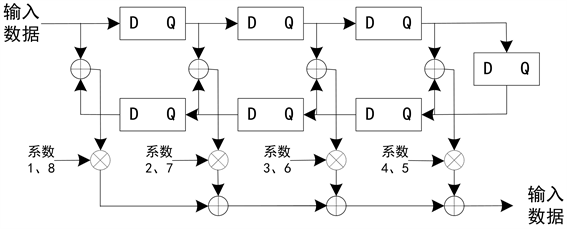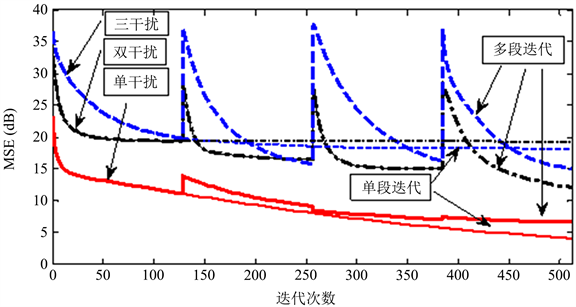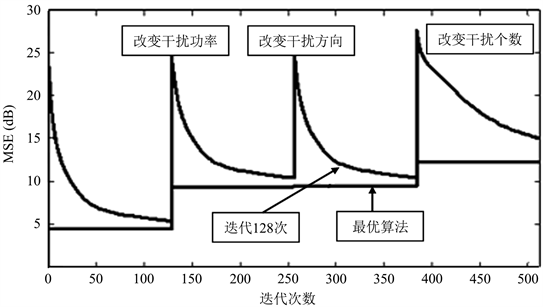#### 期刊菜单

Design and Implementation of Space-Time Joint Anti-Interference and Dimensionality Reduction

Abstract: Aiming at the characteristics of the space-time joint anti-jamming method adopted by the satellite-to-ground measurement and control link, such as the large amount of calculation, the complexity of the system, and the difficulty of adapting to the interference suppression of the complex electromagnetic environment, a combination method of simple iteration method and Gauss-Seidel iteration method is proposed. Dimension processing, dividing the dimension of space-time data processing into several segments, using simple iterative method inside the segment, and Gauss-Seidel iterative method outside the segment to enhance the convergence and parallelism of the algorithm. Through simulation, the feasibility of this combined method is verified.

1. 引言Table 1. Comparison of several algorithms using linear constrained minimum variance criterion

2. 算法设计

$W=\left(I-{R}_{x}\right)W+S=CW+S$ (1)

$I$ 为单位矩阵；

${R}_{x}$ 为空时二维权矢量，为输入的协方差矩阵；

$C=I-{R}_{x}$，为迭代算法的系数矩阵；

$S$$MP×1$ 维的约束向量， $M$ 为阵列天线的阵元数目， $P$ 为时间单元数目。

$\left\{\begin{array}{ll}{w}_{i}^{k+1}=\underset{j=1}{\overset{MP}{\sum }}{c}_{ij}{w}_{j}^{k}+{s}_{i}\hfill & i=1,2,\cdots ,L\hfill \\ {w}_{i}^{k+1}=\underset{j=1}{\overset{L}{\sum }}{c}_{ij}{w}_{j}^{k+1}+\underset{j=L+1}{\overset{MP}{\sum }}{c}_{ij}{w}_{j}^{k}+{s}_{i}\hfill & i=L,L+1,\cdots ,2L\hfill \\ \cdots \hfill & \hfill \\ {w}_{i}^{k+1}=\underset{j=1}{\overset{\left(U-1\right)L}{\sum }}{c}_{ij}{w}_{j}^{k+1}+\underset{j=UL-L+1}{\overset{MP}{\sum }}{c}_{ij}{w}_{j}^{k}+{s}_{i}\hfill & i=UL-L+1,\cdots ,UL\hfill \end{array}$ (2)

$S$$W={\left[{w}_{1},{w}_{2},\cdots ,{w}_{MP}\right]}^{\text{T}}$ 中第i个权值的第k次迭代结果， ${s}_{i}$ 为S的第i个值；

$L$ 为同时进行更新的权值数目，共有 $MP/L=U$ 个；

$U$ 为将空时处理维数 $MP$ 分成的段数。

${\mathrm{log}}_{2}m=MP/m$ (3)

$MP$ 为空时数据处理的维数；

$m$ 为同时并行执行的乘法器个数。

3. 实现方法Figure 1. Structural block diagram of FIR low-pass filter

$X={\left[{x}_{11}{x}_{12}\cdots {x}_{1p}{x}_{21}{x}_{22}\cdots {x}_{2p}\cdots {x}_{M1}{x}_{M2}\cdots {x}_{MP}\right]}^{\text{T}}$ (4)

${x}_{i1}\left(t\right)={x}_{i2}\left(t-\Delta \right)=\cdots ={x}_{iP}\left(t-P\Delta +\Delta \right)i=1,2,\cdots ,M$ (5)

$\Delta \le {T}_{c}/2$$\Delta$ 为时间延迟间隔，为信号的码元时间间隔。

1) 先将空时数据处理的维数MP分成若干段，每一段具有m个数据，并尽量使 ${\mathrm{log}}_{2}m\approx MP/m$

2) 进行m个系数和m个权值的乘法运算，同时，并行开展上一次权值更新时所得结果的累加运算，即求取包含前两次之前的所有 $m/2$ 个加法结果的和；

3) 进行m个系数和m个权值的乘法运算，同时在上述所得加法运算结果的基础上，继续进行加法运算，即求得包含前两次之前的所有 $m/4$ 个加法结果的和；

4) 按照上述规律，继续进行运算，求得包含前两次之前的所有 $m/8$ 个加法结果的和，直到本次权值运算中最后m个乘法结果与m个加法结果的和求取完毕，并且上一次权值运算结果的累加过程完毕为止。

5) 迭代结束后，输出权值。

4. 仿真结果

4.1. 静态干扰仿真结果Figure 2. Performance comparison between single segment data iteration and multi segment data iteration in static state

4.2. 动态干扰仿真结果Figure 3. Comparison of iterative performance of multi segment data under dynamic conditions

5. 结论

  郭艺, 张尔扬, 沈荣骏. 多步迭代降维方法研究[J]. 通信学报, 2007, 28(9): 68-73.  曹建波. GPS接收机降维空时联合处理算法研究[J]. 现代导航, 2014(3): 162-163.  康博, 张新帅, 路明, 吴雅娟, 陈金令. 北斗空时抗干扰降维算法研究[J]. 舰船电子对抗, 2016, 39(6): 73-76.  王霖郁, 杨旭, 项建弘. 基于多域联合处理的MIMO抗干扰技术[J]. 应用科技, 2019, 46(2): 42-46.  王东昱, 马子儒. MIMO系统下的空时正交分组码的研究[J]. 数字技术与应用, 2017(3): 67-68.  王永良, 吴志文, 彭应宁. 适于非均匀杂波环境的空时自适应处理方法[J]. 电子学报, 1999, 27(9): 56-58.  孙晓叔, 皇甫堪, 陈强, 李刚. GPS接收机抗干扰空时自适应滤波方法[J]. 通信学报, 2004, 25(8): 168-173.  董李梅. 一种变步长自适应算法在GPS空时抗干扰中的应用[J]. 通信技术, 2015, 48(3): 295-297.  刘斌, 何广军, 冯有前, 杨科, 许京伟. 静态和差波束匹配的空时自适应处理方法[J]. 西安电子科技大学学报(自然科学版), 2017, 44(3): 138-143.